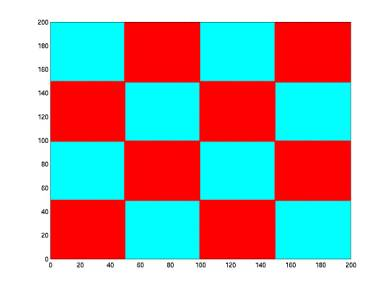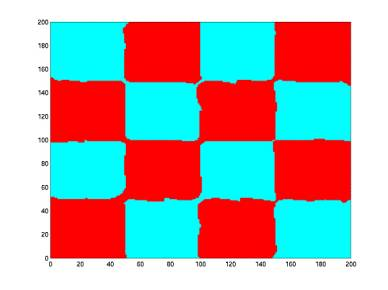Local SVM classification based on Triangulation

Glenn Fung# Abstract

In recent years Support Vector Machines (SVM) have been used widely for classification, regression and in general, supervised and unsupervised learning.

In this work I will concentrate on two-class classification. Briefly described, a support vector machine defines a hyperplane that classifies points by assigning them to one of two disjoint halfspaces. In the case when the set is not linearly separable, the points are  mapped to a higher dimensional space by using a nonlinear kernel. In this new high dimensional space, a linear classifier is appropriate. Two known difficulties that confront large data classification by a nonlinear kernel are:

1-    The possible dependence of the nonlinear separating surface on the entire dataset, which creates unwieldy storage and computational problems that prevent the use of nonlinear kernels for anything but a small dataset.

2- The sheer size of the mathematical programming problem that needs to be solved.

I am proposing a new method to classify points on the plane that addresses the two points mentioned above. In order to achieve this, the dataset has to be divided in different smaller zones (using Delaunay triangulation) where the problem to solve is smaller and thus easier to solve. Once the dataset is divided, each subset is treated as an independent subset where a SVM is applied to find a nonlinear separating surface that is based in a much smaller dataset.

The complete report can be found here:

PowerPoint presentation: